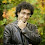### How to easily multiply certain pairs of numbers

I can calculate in about three seconds that 23 x 27 = 621.

Or that 60 x 68 = 4080.

Or that 997 x 1003 = 999,991.

How can I do that so fast? I didn't memorize those numbers, and I didn't use a calculator.

It's easy, if you learn the little trick that I'm about to teach you.

Start with a square number, such as 8 x 8 = 64.

Now subtract some amount from the first number, and add the same amount to the second number, and see what the new product is. Then look at the difference between each product and the original square number:

8 x 8 = 64
7 x 9 = 63 (difference of 1)
6 x 10 = 60 (difference of 4)
5 x 11 = 55 (difference of 9)
4 x 12 = 48 (difference of 16)

Notice a pattern? 1, 4, 9, 16... that's a square number sequence. (4 is 2 x 2, and 9 is 3 x 3, and 16 is 4 x 4.)

Now look at 5 x 11 = 55. The difference between 8 and 5, or between 8 and 11, is 3. The square of 3 is 9. Subtract 9 from 64 and you get... 55.

Cool!

So, how did I use this to do the calculations at the start of this post?

Well, if you memorized your square numbers during recess (while all the cool kids were out kicking soccer balls around and engaging in fisticuffs), you know that 25 x 25 = 625. If you want to calculate 23 x 27, just look at the difference between 23 and 25, which is 2. The square of 2 is 4. Subtract 4 from 625 and you get 621. Therefore, 23 x 27 = 621.

The next one was 60 x 68. The numbers in the middle are 64 x 64. Now I got lucky on this one - I only happen to know it because 64 is a power of 2, i.e. 26 = 64. So the square is double the power, i.e. 26*2 = 212 = 4096.) Difference of 4, squared is 16, subtract from 4096, answer is 4080.

And 1000 x 1000 is easy (a million), so 997 x 1003, difference of 3, squared is 9, subtract from a million, you get 999,991.

I first noticed this sometime in elementary school when practising my times tables, but I couldn't codify it in general terms until I was exposed to algebra in grade 7, which was a revelation to me. I wrote it as:

(x-a)(x+a) = x2-a2

I called this The Sassanian Theorem for two reasons. First, the Pythagorean Theorem was already taken, and my name wasn't Pythagoras anyway. Second, I was a total nerd who was just convinced that this was a revolutionary discovery that will profoundly change the lives of all mathematicians in the future... if I could only publicize it somehow. (It was 1984. I didn't have a blog then.)

Two years later, I learned how to do factoring, and (x-a)(x+a) = x2-a2 didn't seem like anything so clever after all. But it's still a tremendously useful way to quickly multiply two numbers in your head... as long as you know the square of the number midway between them.

I also want to publicly acknowledge that Charles Ledger, my math teacher at the time at Zion Heights Junior High School, smiled and congratulated me on my "discovery" - and refrained from pointing out the obvious similarity to the factoring formula that I would learn two years later. He just made me feel proud and clever. What a great teacher that Mr. Ledger, always encouraging us to think for ourselves!

Hopefully this has been of some use to you. So the next time I tell you that the tax on \$12.99 is \$1.95, don't pull out your calculator to check. Trust the Sassanian Theorem. Thank you. We now return to your regularly scheduled blog, already in progress.you're like rainman or something!Sassan Sanei said…
Yeah, I'm an excellent driver.

Uh oh, 15 minutes to Judge Wapner.